Data visualization in python matplotlib with example

In the previous post, we covered the important DataFrame topic for Data Analysis.In this post, we are covering another important thing in Data Analysis the data exploration using Matplotlib in python.
Luckily, this library is very flexible and has a lot of built-in defaults. As such, you don’t need much to get started: you need to make the necessary imports, prepare the data, and you can start plotting!
Before getting started with matplotlib please check if the necessary installation has been completed.

What are we going to Cover in this topic ?¶

Figures and Subplots¶

• How to Create plotting
• How to show plotting within Notebook.
• Different types of plot you will need for data exploration
• figure - container thats holds all elements of plot(s)
• subplot - appears within a rectangular grid within a figure

Note: Include %matplotlib inline - this will allow to show your graph within notebook.

In :
%matplotlib inline

import pandas as pd
import numpy as np
import matplotlib.pyplot as plt
plt.style.use('ggplot')


In this cell we create a figure with the title, My First Figure. Next, we create two sub plots within the figure. The first two parameters to the subplot function are the number of rows and the number of columns within the rectangular grid of subplots. The third parameter indicates the current subplot, that is the subplot that has been referenced most recently. In contrast to almost every other set of number things in Python, NumbPy and Pandas.

In :
my_first_figure = plt.figure("My First Figure")


<matplotlib.figure.Figure at 0x9f66b00>

In :
subplot_1 = my_first_figure.add_subplot(2, 3, 1)


In :
plt.plot(np.random.rand(50).cumsum(), 'k--')
plt.show()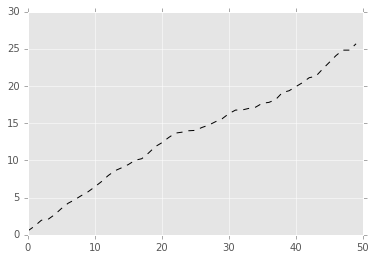Oh! great we have plotted our first plotting.¶

We'll create another random data set, this time we won't use the cumulative sum function. Essentially the data will be a scatter plot. We will use green circles indicated by G for green and O for circles to illustrate the plot. In plot number two the X axis ranges from zero to 50, corresponding to the 50 data points that we randomly generated. The Y axis ranges from zero to one, let's illustrate this plot.

Scatterplot in Matplotlib¶

In :
subplot_2 = my_first_figure.add_subplot(2, 3, 2)
plt.plot(np.random.rand(50), 'go')


Out:

[<matplotlib.lines.Line2D at 0xa312978>]

In :
subplot_6


Out:

<matplotlib.axes._subplots.AxesSubplot at 0xa3204a8>

In :
plt.show()


We will develop random tempreature data to work with some great exploration and plotting.In the next section we will look plot and sub plot.¶
In :
data_set_size = 15
low_mu, low_sigma = 50, 4.3
low_data_set = low_mu + low_sigma * np.random.randn(data_set_size)
high_mu, high_sigma = 57, 5.2
high_data_set = high_mu + high_sigma * np.random.randn(data_set_size)

days = list(range(1, data_set_size + 1))


We'll begin with a data_set_size of 15. The final statement in this cell uses an assignment statement to create a python list, named days. We wrap python's list function around python's range function to create a list of values from one to the size of our data set. Execute this cell. We'll begin by displaying a plot with only the low temperature data.

In :
plt.plot(days, low_data_set)
plt.show()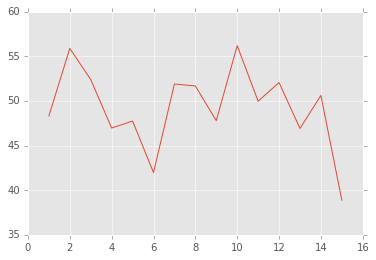Using these parameters, we are telling Matplotlib to use days along the horizontal axis, and to use temperatures upon the vertical axis. By corresponding, I mean that day number one corresponds to the first data point in the data set, and day number two corresponds to the second data point in the data set, and so forth. In this cell, we'll plot both the high and low data sets on the same plot.

In :
plt.plot(days, low_data_set,
days, high_data_set)
plt.show()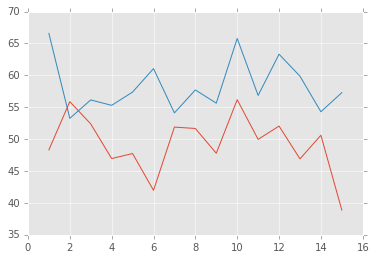In :
plt.plot(days, low_data_set,
days, low_data_set, "vm",
days, high_data_set,
days, high_data_set, "^k")
plt.show()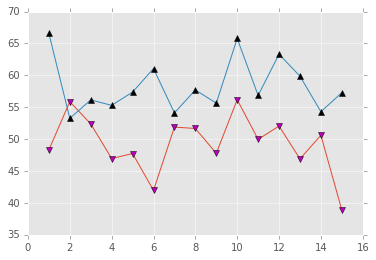Here, it's relatively easy to see that the high temperature data set is illustrated in blue, and the low temperature data set is illustrated in red. Let's enhance this plot by adding magenta-colored, downward-pointing triangles; that's the v for the downward-pointing triangles, and m for the color, and black, k, upward-pointing triangles for the high_data_set. We'll add a label on the x-axis, we'll add a label on the y-axis, and we'll include a table for the plot.

In :
plt.plot(
days, high_data_set, "^k")
plt.show()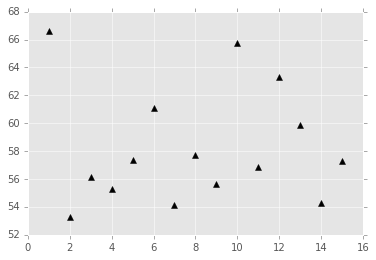In [ ]:

In :
plt.plot(days, low_data_set,
days, low_data_set, "vm",
days, high_data_set,
days, high_data_set, "^k")
plt.xlabel('Day')
plt.ylabel('Temperature: degrees Farenheit')
plt.title('Randomized temperature data')
plt.show()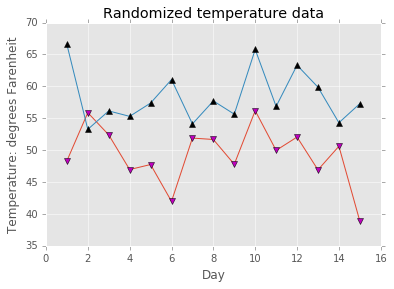In :
plt.plot(days, low_data_set,

days, high_data_set
)
plt.xlabel('Day')
plt.ylabel('Temperature: degrees Farenheit')
plt.title('Randomized temperature data')
plt.show()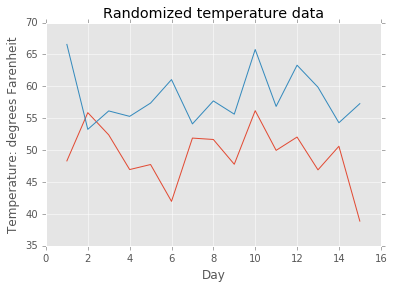In :
plt.plot(
days, high_data_set, "^k")
plt.xlabel('Day')
plt.ylabel('Temperature: degrees Farenheit')
plt.title('Randomized temperature data')
plt.show()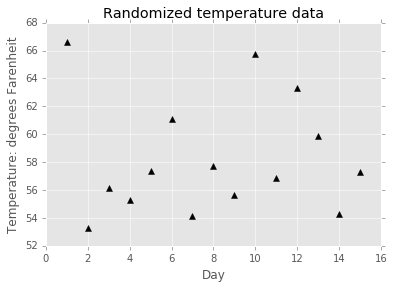In :
t1 = np.arange(0.0, 2.0, 0.1)
t2 = np.arange(0.0, 2.0, 0.01)

# note that plot returns a list of lines.  The "l1, = plot" usage
# extracts the first element of the list into l1 using tuple
# unpacking.  So l1 is a Line2D instance, not a sequence of lines
l1, = plt.plot(t2, np.exp(-t2))
l2, l3 = plt.plot(t2, np.sin(2 * np.pi * t2), '--go', t1, np.log(1 + t1), '.')
l4, = plt.plot(t2, np.exp(-t2) * np.sin(2 * np.pi * t2), 'rs-.')

plt.legend((l2, l4), ('oscillatory', 'damped'), loc='upper right', shadow=True)
plt.xlabel('time')
plt.ylabel('volts')
plt.title('Damped oscillation')
plt.show()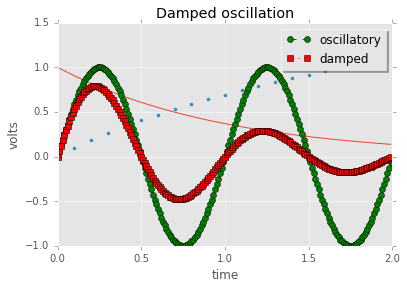We can use these references to lines to create a legend for the plot. The first parameter is a couple of lines that we want to use in the legend. The second parameter is a couple of strings for the legend. The third parameter is named, and it indicates the location for the legend. The fourth parameter is also named, and it includes a styling parameter. When we execute this cell, we see the plot. This is a relatively complex plot.

How to plot a histogram in Matplotlib?¶

The below example develops a random dataset which we will use for plotting a histogram.

In :
mu, sigma = 100, 15
data_set = mu + sigma * np.random.randn(10000)

# the histogram of the data
n, bins, patches = plt.hist(data_set, 50, normed=1, facecolor='y', alpha=0.75)

plt.xlabel('Smarts')
plt.ylabel('Probability')
plt.title('Histogram of IQ')
plt.text(60, .025, r'$\mu=100,\ \sigma=15$')
plt.axis([40, 160, 0, 0.03])
plt.grid(True)
plt.show()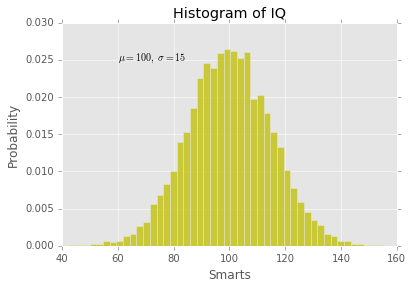Available Colors:


code       color
________________________
'k'         black
'b'         blue
'c'         cyan
'g'         green
'm'         magenta
'r'         red
'w'         white
'y'         yellow



How to plot a Ticked Plot ?¶

In :
number_of_data_points = 1000

my_figure = plt.figure()
my_data_set = np.random.rand(number_of_data_points).cumsum()
subplot_1.plot(np.random.rand(number_of_data_points).cumsum())

number_of_ticks = 5
ticks = np.arange(0, number_of_data_points, number_of_data_points//number_of_ticks)
subplot_1.set_xticks(ticks)

labels = subplot_1.set_xticklabels(['one', 'two', 'three', 'four', 'five'], rotation=45, fontsize='small')

subplot_1.set_title ("My First Ticked Plot")
subplot_1.set_xlabel ("Groups")

subplot_1.grid(True)
gridlines = subplot_1.get_xgridlines() + subplot_1.get_ygridlines()
for line in gridlines:
line.set_linestyle(':')

plt.show()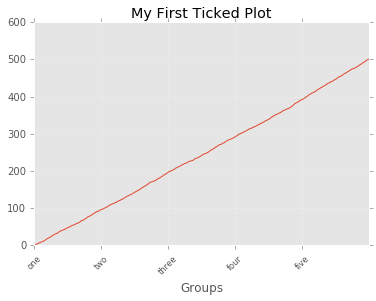Plot Annotations

In the below example we will look to work with annotations you will be using while plotting.Look below some of the amazing plotting example

In :
number_of_data_points = 10

my_figure = plt.figure()
subplot_1.plot(np.random.rand(number_of_data_points).cumsum())

subplot_1.text (1, 0.5, r'an equation: $E=mc^2$', fontsize=18, color='red')
subplot_1.text (1, 1.5, "Hello, Mountain Climbing!", family='monospace', fontsize=14, color='green')

# see: http://matplotlib.org/users/transforms_tutorial.html
# transform=subplot_1.transAxes; entire axis between zero and one
subplot_1.text(0.5, 0.5, "We are centered, now", transform=subplot_1.transAxes)

subplot_1.annotate('shoot arrow', xy=(2, 1), xytext=(3, 4),
arrowprops=dict(facecolor='red', shrink=0.05))

plt.show()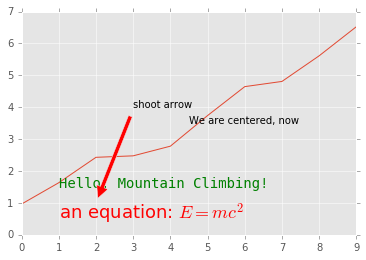In :
x = np.arange(0, 10, 0.005)
y = np.exp(-x/2.) * np.sin(2*np.pi*x)

fig = plt.figure()
ax.plot(x, y)
ax.set_xlim(0, 10)
ax.set_ylim(-1, 1)

xdata, ydata = 5, 0
xdisplay, ydisplay = ax.transData.transform_point((xdata, ydata))

bbox = dict(boxstyle="round", fc="0.8")
arrowprops = dict(
arrowstyle = "->",

offset = 72
ax.annotate('data = (%.1f, %.1f)'%(xdata, ydata),
(xdata, ydata), xytext=(-2*offset, offset), textcoords='offset points',
bbox=bbox, arrowprops=arrowprops)

disp = ax.annotate('display = (%.1f, %.1f)'%(xdisplay, ydisplay),
(xdisplay, ydisplay), xytext=(0.5*offset, -offset),
xycoords='figure pixels',
textcoords='offset points',
bbox=bbox, arrowprops=arrowprops)

plt.show()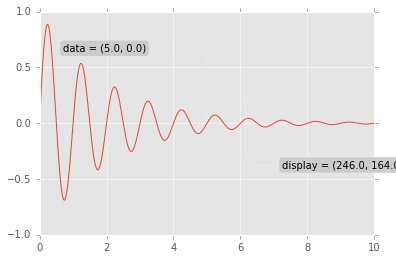In :
fig = plt.figure()
for i, label in enumerate(('A', 'B', 'C', 'D')):
ax.text(0.05, 0.95, label, transform=ax.transAxes,
fontsize=16, fontweight='bold', va='top')

plt.show()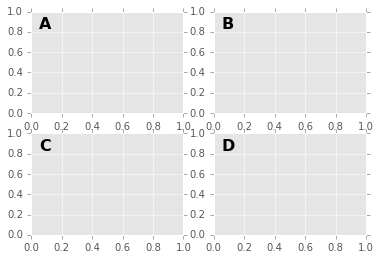Amazing! we have completed looking some amazing plotting using matplotlib. In the next post we'll be looking to plot with Dataframe.Till that feel free to post your doubt in below comment section and share it on social media.

In [ ]:

Alll the world is a laboratory to the inquiring mind! Join UsRahul11 said…
Awesome Post! Neat Explanation.JackMusk said…